# norse.torch.utils.plot package¶

A module for plotting event-based data and module information such as weights and neuron parameters.

norse.torch.utils.plot.plot_heatmap_2d(data, axes=None, show_colorbar=False, **kwargs)[source]

Plots a heatmat of two-dimensional data

Example

>>> data = torch.randn(28, 28)
>>> plot_heatmap_2d(data)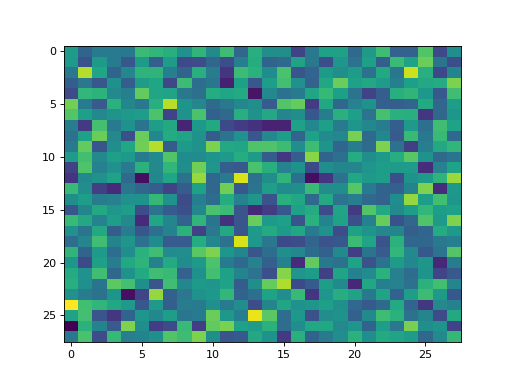Parameters
• data (torch.Tensor) – A tensor of data to plot in two dimensions (typically spikes with time in the first dimension and neuron id in the second).

• axes (matplotlib.axes.Axes) – The matplotlib axis to plot on, if any. Defaults to matplotlib.pyplot.gca()

• show_colorbar (bool) – Show a colorbar (True) or not (False).

• kwargs – Specific key-value arguments to style the figure fed to the matplotlib.pyplot.imshow() function.

Returns
norse.torch.utils.plot.plot_heatmap_3d(spikes, show_colorbar=False, **kwargs)[source]

Plots heatmaps for some activity in several layers. Expects a named tensor with names=(‘L’, ‘X’, ‘Y’). Instead of using the matplotlib.pyplot.imshow() matplotlib function, we make use of the matplotlib.pyplot.scatter() function to disperse points in 3d.

Example

>>> import torch
>>> from norse.torch.utils.plot import plot_heatmap_3d
>>> data = torch.randn(4, 28, 28, names=('L', 'X', 'Y'))
>>> plot_heatmap_3d(data)
>>> plt.show()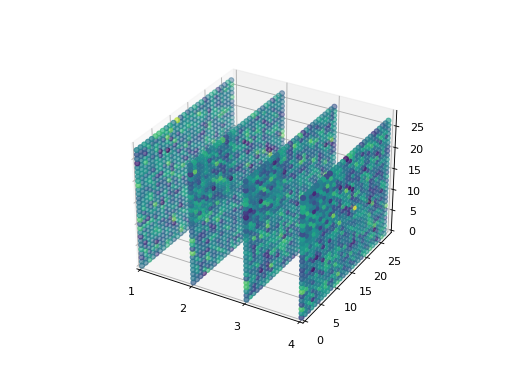Parameters
• spikes (torch.NamedTensor) – A tensor named with four dimensions: T (time), L (layer), X, Y. Expected to be in the range $$[0, 1]$$.

• show_colorbar (bool) – Show a colorbar (True) or not (False).

• kwargs – Specific key-value arguments to style the figure fed to the matplotlib.pyplot.scatter() function.

Returns

An matplotlib.axes.Axes object

norse.torch.utils.plot.plot_histogram_2d(spikes, axes=None, **kwargs)[source]

Plots a histogram of 1-dimensional data.

Example

>>> cell = LIF()
>>> data = torch.ones(10, 10) + torch.randn(10, 10)
>>> spikes, state = cell(data)
>>> plot_histogram_2d(state.v)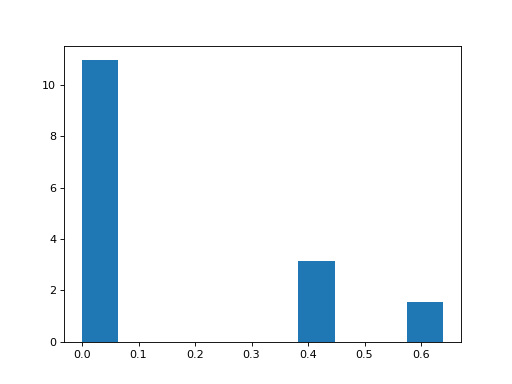Parameters
• data (torch.Tensor) – A tensor of single-dimensional data.

• axes (matplotlib.axes.Axes) – The matplotlib axis to plot on, if any. Defaults to matplotlib.pyplot.gca()

• kwargs – Specific key-value arguments to style the figure fed to the matplotlib.pyplot.hist() function.

Returns
norse.torch.utils.plot.plot_izhikevich(behavior, current=1, time_print=250, time_current=20, timestep_print=0.1)[source]

Computes and plots a 2D visualisation of the behavior of an Izhikevich neuron model. By default, the time window is 250ms with a time step of 0.1ms

Example :
>>> import torch
>>> from norse.torch import tonic_spiking
>>> from norse.torch.utils.plot import plot_izhikevich
>>> plot_izhikevich(tonic_spiking)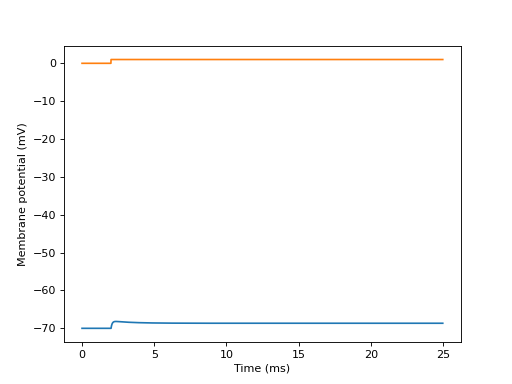Arguments :

behavior (IzhikevichSpikingBehavior) : behavior of an Izhikevich neuron current (float) : strengh of the input current, defaults to 1 time_print (float) : size of the time window for simulation (in ms) time_current (float) : time at which the input current goes from 0 to current (in ms) timestep_print (float) : timestep of the simulation (in ms)

norse.torch.utils.plot.plot_scatter_3d(spikes, axes=None, show_colorbar=True, **kwargs)[source]

Plots spike activity in time. If multiple layers are given, the layers will be shown in subplots. Expects a named tensor in three dimensions (L, X, Y) or four, with time (T, L, X, Y).

Example

>>> distribution = torch.distributions.bernoulli.Bernoulli(torch.tensor([0.02]))
>>> data = distribution.sample(sample_shape=(3, 100, 10, 10)).squeeze()
>>> data.names = ('L', 'T', 'X', 'Y')
>>> plot_scatter_3d(data)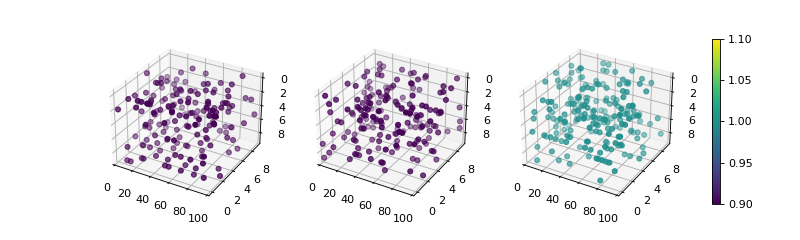Parameters
• spikes (torch.NamedTensor) – A tensor named with four dimensions: T (time), L (layer), X, Y. Expected to be in the range $$[0, 1]$$.

• axes (List[plt.Axes]) – A list of Axes that should have the same length as the L dimension in the spike tensor. Defaults to None, which will generate a grid for you.

• show_colorbar (bool) – Show a colorbar (True) or not (False).

• kwargs – Specific key-value arguments to style the figure fed to the matplotlib.pyplot.scatter() function.

Returns

A list of matplotlib.axes.Axes

norse.torch.utils.plot.plot_spikes_2d(spikes, axes=None, **kwargs)[source]

Plots a 2D diagram of spikes. Works similar to the plot_heatmap_2d() but in black and white.

Example

>>> import torch
>>> from norse.torch import LIF
>>> from norse.torch.utils.plot import plot_spikes_2d
>>> spikes, _ = LIF()(torch.randn(200, 10))
>>> plot_spikes_2d(spikes)
>>> plt.show()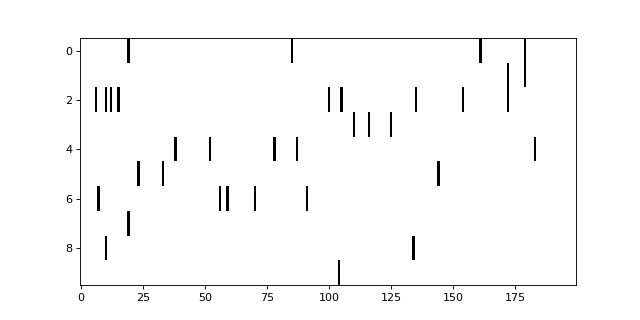Parameters
• spikes (torch.Tensor) – A tensor of spikes from a single layer in two dimensions.

• axes (matplotlib.axes.Axes) – The matplotlib axis to plot on, if any. Defaults to matplotlib.pyplot.gca()

• kwargs – Specific key-value arguments to style the figure fed to the matplotlib.pyplot.imshow() function.

Returns

An matplotlib.axes.Axes object